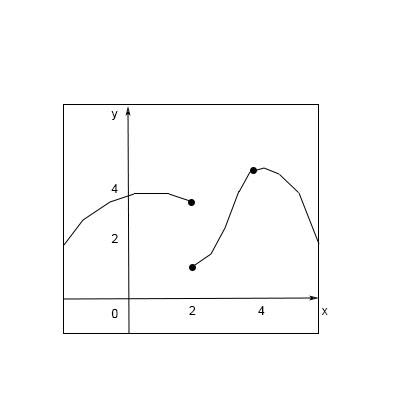# Use the given graph of f to state the value of each quantity, if it exists. (If an answer does...

## Question:

Use the given graph of f to state the value of each quantity, if it exists. (If an answer does not exist , enter DNE.)

a. {eq}\lim \limits {x \to 2 ^-} f(x) {/eq}

b. {eq}\lim \limits {x \to 2 ^+} f(x) {/eq}

c. {eq}\lim \limits {x \to 2 } f(x) {/eq}

d. {eq}f(2) {/eq}

e. {eq}\lim \limits {x \to 4} f(x) {/eq}

f. {eq}f(4) {/eq}## Limits of a Function:

The limit in one of the very important concepts in calculus, it is used to find the value of the graph of the function at the point where it is broken or discontinuous. So limits exist if we can find the approaching value of the function.

Using the above graph, lets find

a. {eq}\lim \limits {x \to 2 ^-} f(x)=3 {/eq}

b. {eq}\lim \limits {x \to 2 ^+} f(x)=1 {/eq}

c. {eq}\lim \limits {x \to 2 ^-} f(x) \neq \lim \limits {x \to 2 ^+} f(x)\\ \lim \limits {x \to 2 } f(x)=DNE {/eq}

d. {eq}f(2)=1, 3 {/eq}

e. {eq}\lim \limits {x \to 4} f(x)=4 {/eq}

f. {eq}f(4)=4 {/eq}